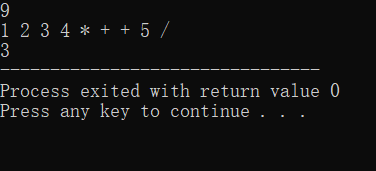# 后缀式转换为中缀式计算结果

## 源代码

#include<iostream>
using namespace std;
#define maxSize 50
int op(int a,char Op,int b)//本函数是运算函数，用来完成算式“a Op b”的运算
{
if(Op=='+')
return a+b;
if(Op=='-')
return a-b;
if(Op=='*')
return a*b;
if(Op=='/'){
if(b==0){
cout<<"ERROR";
return 0;
}else{
return a/b;
}
}
}
int com(char exp[])//后缀式计算函数
{
int i,a,b,c;
int stack[maxSize];
int top=-1;
char Op;
for(i=0;exp[i]!='\0';++i){
if(exp[i]>='0'&&exp[i]<='9')
stack[++top]=exp[i]-'0';//因为数组是字符数组，0-9数字才可以用这个方法
else{
Op=exp[i];
b=stack[top--];//后入的是b，先取b
a=stack[top--];
c=op(a,Op,b);//调用运算函数
stack[++top]=c;
}
}
return stack[top];
}

int main()
{
int n;
cin>>n;
char exp[maxSize];
for(int i=0;i<n;++i){
cin>>exp[i];
}
cout<<com(exp);
return 0;
}


## 执行结果©️2019 CSDN 皮肤主题: 1024 设计师: 上身试试